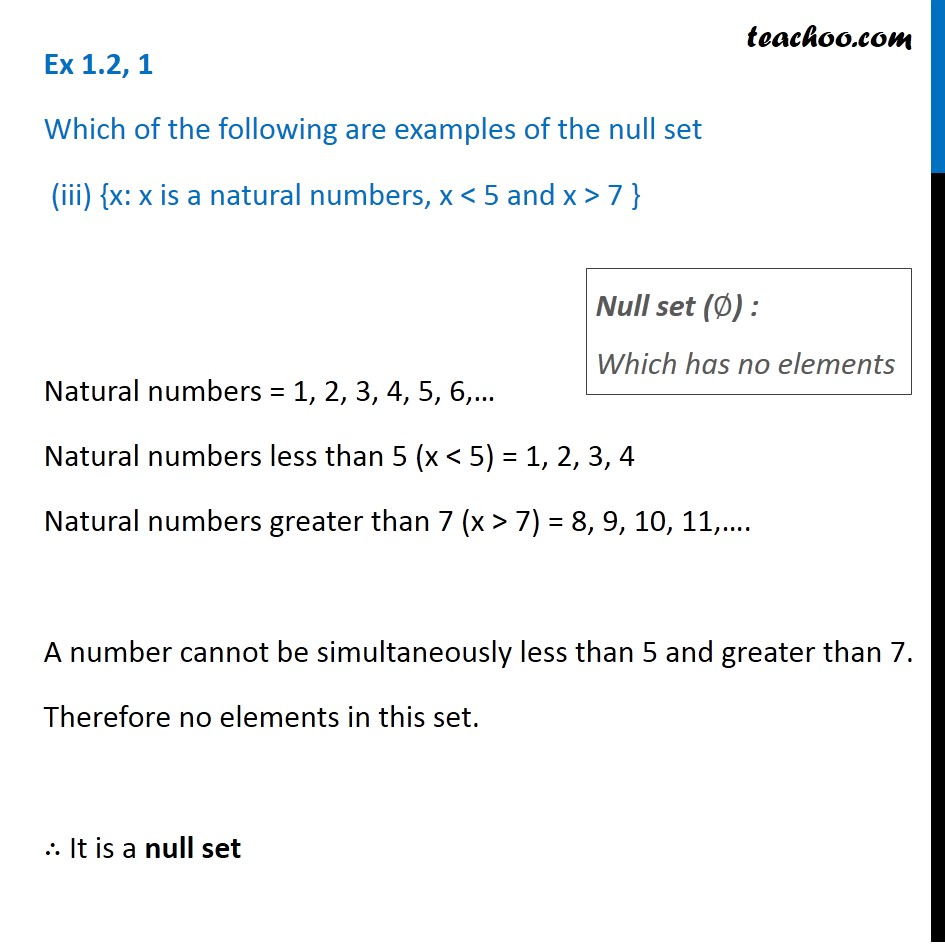Ex 1.2

Chapter 1 Class 11 Sets
Serial order wiseGet live Maths 1-on-1 Classs - Class 6 to 12

### Transcript

Ex 1.2, 1 Which of the following are examples of the null set (iii) {x: x is a natural numbers, x < 5 and x > 7 } Natural numbers = 1, 2, 3, 4, 5, 6,… Natural numbers less than 5 (x < 5) = 1, 2, 3, 4 Natural numbers greater than 7 (x > 7) = 8, 9, 10, 11,…. A number cannot be simultaneously less than 5 and greater than 7. Therefore no elements in this set. ∴ It is a null set Null set (∅) : Which has no elements